Theory.org » fracdyn » burningship » Symmetry in the Burning Ship to higher powers

Symmetry in the Burning Ship to higher powers

The general form of the Burning Ship used here is the iterated function z -> (|re(z)|+i*|im(z)|)n+c,
where z is a point in the complex plane x+i*y, and re(z)=x, im(z)=y.
Notice how the even powers are asymmetric, and the odd powers are symmetric about the diagonal.
This symmetry seems to be a result of the way that the function factors out.
The terms in the odd powers are more symmetrical.
for powers of 4n-1, the point u,v will be the same as v,u.
for powers of 4n-3, the point u,v will be the same as -v,u.
Burning Ship Home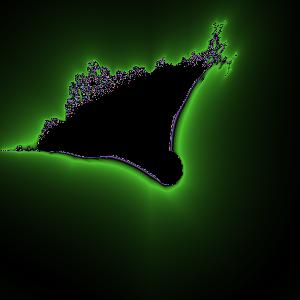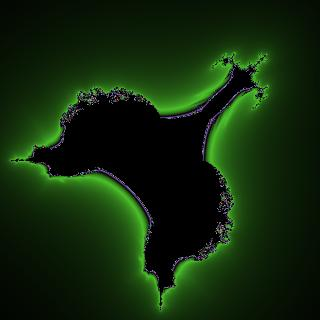(|x|+i*|y|)2+c
Cubic (sometimes called the Bird of Prey)
(|x|+i*|y|)3+c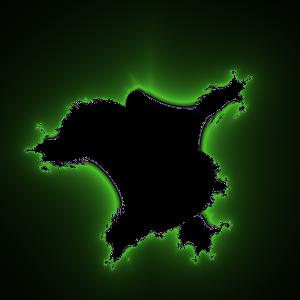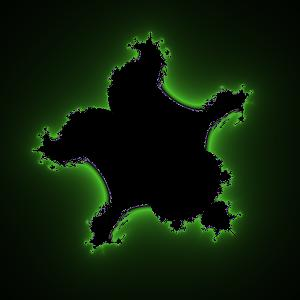Quartic
(|x|+i*|y|)4+c
Quintic
(|x|+i*|y|)5+c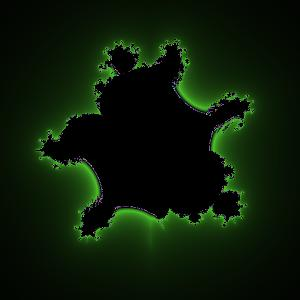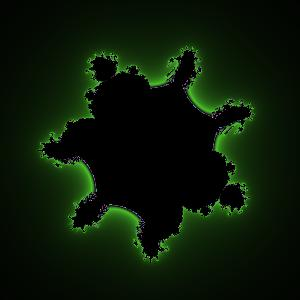Sixth Power
(|x|+i*|y|)6+c
Seventh Power
(|x|+i*|y|)7+c